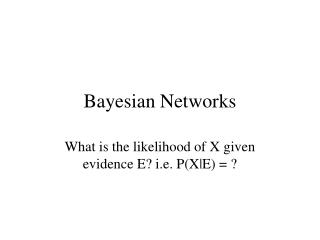DownloadDownload PresentationBayesian Networks

# Bayesian Networks

Télécharger la présentation## Bayesian Networks

- - - - - - - - - - - - - - - - - - - - - - - - - - - E N D - - - - - - - - - - - - - - - - - - - - - - - - - - -
##### Presentation Transcript

1. Bayesian Networks What is the likelihood of X given evidence E? i.e. P(X|E) = ?

2. Issues • Representational Power • allows for unknown, uncertain information • Inference • Question: What is Probability of X if E is true. • Processing: in general, exponential • Acquisition or Learning • network: human input • probabilities: data+ learning

3. Bayesian Network • Directed Acyclic Graph • Nodes are RV’s • Edges denote dependencies • Root nodes = nodes without predecessors • prior probability table • Non-root nodes • conditional probabilites for all predecessors

4. Bayes Net Example: Structure Earthquake Burglary Alarm Mary Calls John Calls

5. Probabilities Structure dictates what probabilities are needed P(B) = .001 P(-B) = .999 P(E) = .002 P(-E) = .998 etc. P(A|B&E) = .95 P(A|B&-E) = .94 P(A|-B&E) = .29 P(A|-B&-E) = .001 P(JC|A) = .90 P(JC|-A) = .05 P(MC|A) = .70 P(MC|-A) = .01

6. Joint Probability yields all • Event = fully specified values for RVs. • Prob of event: P(x1,x2,..xn) = P(x1|Parents(X1))*..P(xn|Parents(Xn)) • E.g. P(j&m&a&-b&-e) = P(j|a)*P(m|a)*P(a|-b^-e)*P(-b)*P(-e) = .9*.7*.001*.999*..998 = .00062. • Do this for all events and then sum as needed. • Yields exact probability (assumes table right)

7. Many Questions • With 5 boolean variables, joint probability has 2^5 entries, 1 for each event. • A query corresponds to the sum of a subset of these entries. • Hence 2^2^5 queries possibles. – 4 billion possible queries.

8. Probability Calculation Cost • With 5 boolean variables need 2^5 entries. In general 2^n entries with n booleans. • For Bayes Net, only need tables for all conditional probabilities and priors. • If max k inputs to a node, and n RVs, then need at most n*2^k table entries. • Data and computation reduced.

9. Example Computation Method: transform query so matches tables Bold = in a table P(Burglary|Alarm) = P(B|A) = P(A|B)*P(B)/ P(A) P(A|B) = P(A|B,E)*P(E)+P(A|B,~E)*P(~E). Done. Plug and chug.

10. Query Types • Diagnostic: from effects to causes • P(Burglary | JohnCalls) • Causal: from causes to effects • P(JohnCalls | Burglary) • Explaining away: multiple causes for effect • P(Burglary | Alarm and Earthquake) • Everything else

11. Approximate Inference • Simple Sampling: logic sample • Use BayesNetwork as a generative model • Eg. generate million or more models, via topological order. • Generates examples with appropriate distribution. • Now use examples to estimate probabilities.

12. Logic Sampling: simulation • Query: P(j&m&a&-b&-e) • Topological sort Variables, i.e • Any order that preserves partial order • E.g B, E, A, MC, JC • Use prob tables, in order to set values • E.g. p(B = t) = .001 => create a world with B being true once in a thousand times. • Use value of B and E to set A, then MC and JC • Yields (1 million) .000606 rather than .00062 • Generally huge number of simulations for small probabilities.

13. Sampling -> probabilities • Generate examples with proper probability density. • Use the ordering of the nodes to construct events. • Finally count to yield an estimate of the exact probability.

14. Sensitivity Analysis:Confidence of Estimate • Given n examples and k are heads. • How many examples needed to be 99% certain that k/n is within .01 of the true p. • From statistic: Mean = np, Variance = npq • For confidence of .99, t = 3.25 (table) • 3.25*sqrt(pq/N) < .01 => N >6,400. • But correct probabilities not needed, just correct ordering.

15. Lymphoma DiagnosisPathFinder systems • 60 diseases, 130 features • I: rule based, performance ok • II: used mycin confidence, better • III: Do Bayes Net: best • IV: Better Bayes Net: (add utility theory) • outperformed experts • solved the combination of expertise problem

16. Summary • Bayes nets easier to construct then rule-based expert systems • Years for rules, days for random variables and structure • Probability theory provides sound basis for decisions • Correct probabilities still a problem • Many diagnostic applications • Explanation less clear: use strong influences